# iFit: plotting iData objects

Commands we use in this page: plot, subplot, xlabel, xlim, title, saveas, methods

The iData objects can be plotted. They then show the Signal, usually divided by the Monitor, as a function of the defined Axes (or default ones when not defined). A way to plot the raw Signal is to set the Monitor to 1 or 0.

You can of course display the object information with the disp method:
`>> a=load(iData, [ ifitpath 'Data/sv1850.scn' ])>> disp(a)`
or edit its values in a spreadsheet:
`>> edit(a)`

### Plotting objects in a single pane (plot)

The main plot method for iData objects is plot. It works for objects up to ndims(a)<=3. Further dimensionality objects may be visualized by means of projections and slices, as seen below. The quantity which appears onto plots is the Signal/Monitor. In order to vizualize the raw Signal, set Monitor to 1 with e.g.
`>> a=load(iData, [ ifitpath 'Data/sv1850.scn' ])>> plot(a)>> old_mon=getalias(a,'Monitor');  % save the current Monitor alias if you wish to restore it afterwards>> setalias(a,'Monitor',1);>> figure; plot(a)`
The plot windows offer 'camera ready' quality rendering using openGL. Standard tools are available zoom, edit, pan, export image, rotate, explore data points with a cursor, customize colors, ...
The plot style can be tuned to mesh, contour, line, scatter, surface, volumes, waterfall, ...

#### Plots for 1D objects

Let us import a simple 1D (vector Signal=f(x) ) data set into an iData object, and plot it:
`>> a=load(iData, [ ifitpath 'Data/sv1850.scn' ])>> plot(a);`

A standard Matlab figure appears, with the Signal and the Axis of rank 1. The curve itself can be right-cliked in order to pop-up a contextual menu which provides (see left image):
• an information dialog about the object (see right image above) and the iFit/iData library version,
• the possibility to duplicate the view into an other window,
• simple ways to toggle a grid and error bar display,
• simple way to toggle linear or log scale for the Signal
• a 'Reset view' item to re-plot the initial object view.
Tip: in case the contextual menu item linear/log toggle does not satisfy your expectations, you may try to directly plot the log of the object with (see Math operators)
`>> plot( log10(a) );`
As for any Matlab window, it may be edited using the arrow tool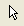which allows to double-click the labels, title, the axes and the curve in order to change e.g. the fonts, the text to show, the colors.
The zoom tool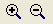is also pretty useful (hey ?). To revert to the full view, double click on the figure, or select the contextual menu item 'Reset view'.

The Matlab documentation about Graphics/Plots and Plotting Tools/Figures, Plots, and Graphs provides a full description of all the tools available in Matlab plot windows. Most of the functionality can also be accessed from the Figure menus. We encourage you to read all this, especially late at night in winter.

Additional arguments can be given to plot in order to specify the line style and color, such as in the standard Matlab plot function, e.g. plot(a,'r--') to draw a red dashed line. The special style 'hide_errorbars' will not show error bars, but they can be made visible from the contextual menu. The h=plot(...) function returns a Matlab graphics handle (this is usually a number). It may be used to perform further access and modification to the view.

#### Plots for 2D objects

Similarly as for 1D objects, plots can be generated for 2D objects Signal=f(x,y), and show them up as surfaces:
`>> a=load(iData, [ ifitpath 'Data/ILL_D10.dat' ])>> plot(a);`
which results in a surface plot as shown aside.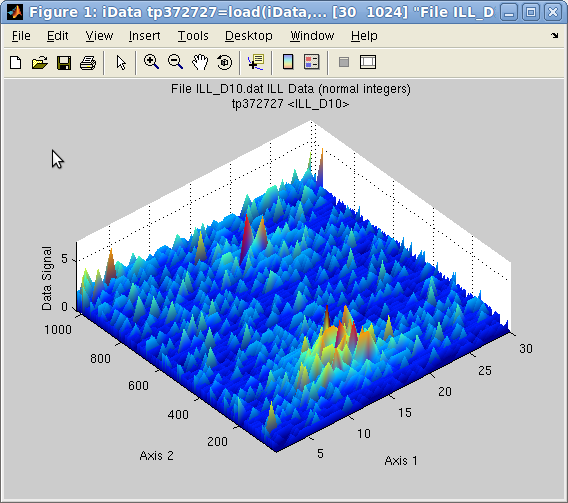Plot 2D: plot(a) with smooth view

Alternate rendering can be obtained with the plot options:
`>> a=iData(peaks);>> plot(a);           % a surface, same as surf(a)>> plot(a,'mesh');    % a wired mesh, same as mesh(a)>> plot(a,'contour'); % contour plot, same as contour(a)>> plot(a,'contourf');% contour plot with filled regions, same as contourf(a)>> plot(a,'surfc');   % a surface with contour plot below, same as surfc(a)>> plot(a,'surfl');   % a surface with light, same as surfl(a)>> plot(a,'plot3');   % a surface made of lines side by side, same as plot3(a)>> plot(a,'scatter3');% a surface made of colored points, same as scatter3(a)>> plot(a,'waterfall');% a surface made of colored points, same as waterfall(a)`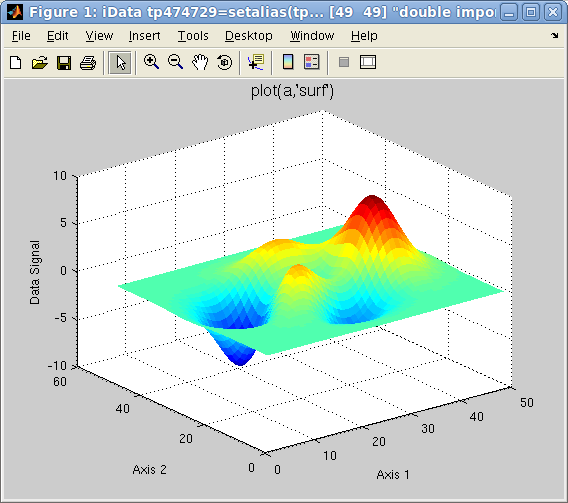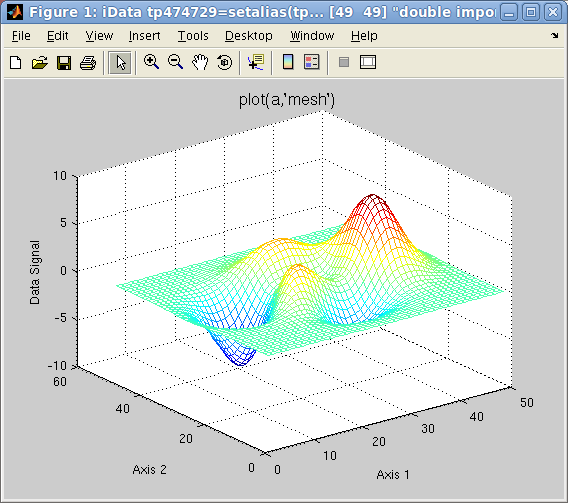Plot 2D: left=plot(a), center=mesh(a), right=surfc(a)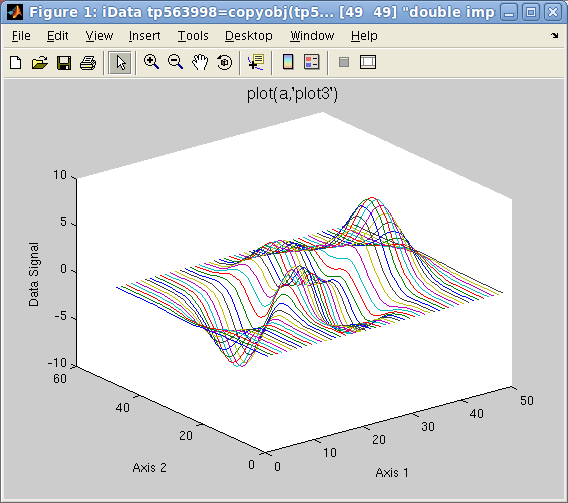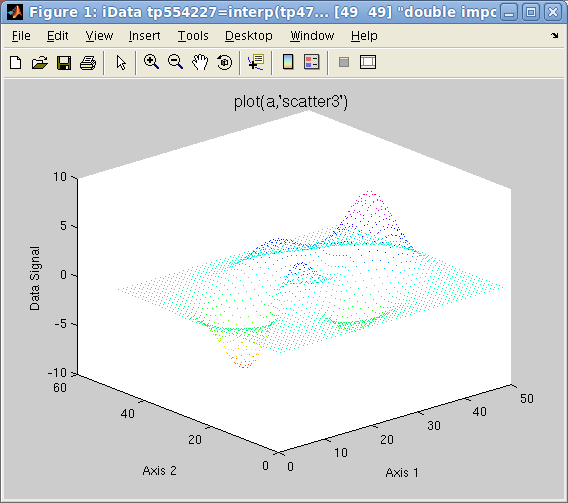Plot 2D: left=plot3(a), right=scatter3(a)

Other plot types can be requested with keywords in the second argument to plot:
• contour3 (a countour in 3D)
• surfl (surface with light)
• pcolor (a flat matrix/image view, may be advantageously coupled with the hide_axis option)
• stem3 (single points which show elevation from basal plane)
##### Data shown as an image (flat)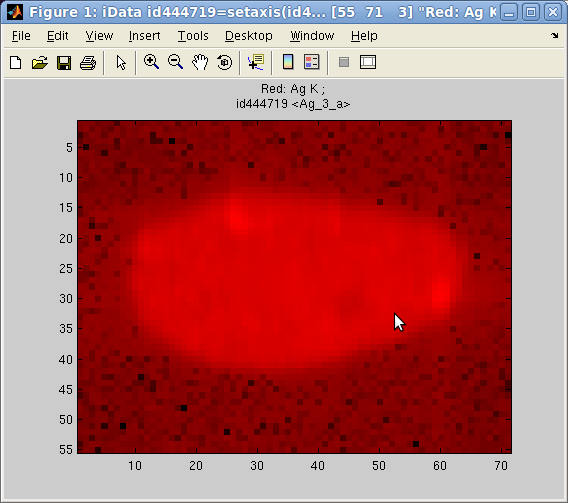In addition, the image(r,g,b) plotting method allows to send up to 3 objects onto the RGB color channels, and display them as a flat image (when using option='hide_axis') :
`>> a = iData([ ifitpath 'Data/Ag_3_a.edf' ])>> h = pcolor(a);			% full color image>> h = image(log(a), 'hide_axes');	% red   (1st) channel image>> h = image([], a, 'hide_axes');	% green (2nd) channel image>> h = image([],[], a, 'hide_axes');	% blue  (3rd) channel image`
Some of the RGB channels can be left empty to suppress one color. Each object is re-scaled in the [0 1] range to use the full channel colors. However, when using the option 'norm' keyword, the scaling is made global to all images, enabling comparison of relative intensity between channel contents.
An other possibility to supperpose different contributions with separated colors is to use the colormap method below.
##### Mapping an object onto a surface (CData)
When a surface has been already plotted, either from the Matlab standard surf or the iData plotting methods presented above, the color shown on surfaces are usually the value of the data itself.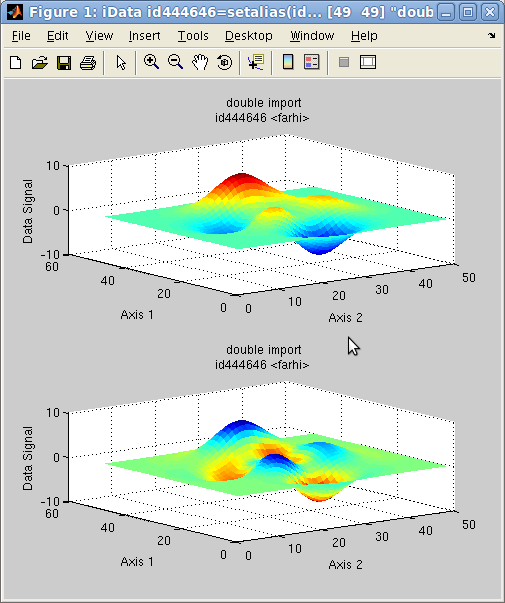It is possible to map the values of any iData object onto a surface. When the iData object does not match the surface dimensions, a rescaling is automatically performed. This is achieved with the caxis method.
In the following example, we plot a surface, and then map the colors shown on that surface (aka CData) with the Laplacian of the surface (curvature):
`>> a = iData(peaks); >> h = subplot([ a a ]); >> caxis(del2(a),h(2));	% we map the Laplacian del2 onto the second plot`
The initial object is shown in the upper panel, whereas the re-mapped object is shown below. The blue regions indicate a negative curvature (top of hills), and the red ones indicate a positive curvature (bottom of hills).
##### Having separate colors in plot of arrays
It is possible to associate one colormap (that is a set of colors glued onto a surface) for each object displayed in a same coordinate frame/window. The colormap function should then be used. It supports the same options as plot, but colormaps can be specified in the list of arguments. When ommitted, a set of predefined colormaps is chosen for each object.
`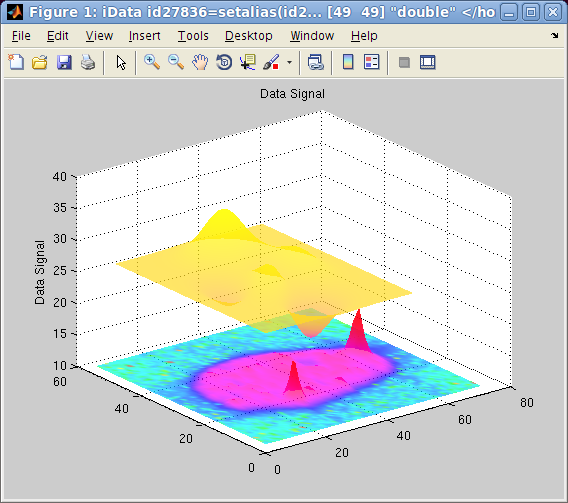`
This plotting method is particularly pretty looking when using the 'shifted' option, which then separate objects one from the other so that they can be well identified on the figure. Choosing colormaps which are well separated in color scales allow to visualize different contributions, just like the image plotting method.
`>> a = iData([ ifitpath 'Data/Ag_3_a.edf' ]);>> b = iData(peaks);>> colormap(a/10000,hsv,b,spring,'shifted transparent interp');`
The first object is set to scale, and plotted with the hsv color set. The second object is shown with the spring color set. Adding the 'log' keyword to the options will use the log of the signal for the color scale, in order to enhance low signals.
It is possible, by right-clicking on the surface or the axis lines, to pop-up a contextual menu which provides the following operations:
• an information dialog about the object (see right image above) and the iFit/iData library version,
• the possibility to duplicate the view into an other window,
• simple way to toggle a grid
• the possibility to smooth the surface appearance, add light and make it semi-transparent. Consecutive light and transparency selections enhance the rendering
• simple way to toggle linear or log scale for the Signal
• simple way to toggle a perspective rendering
• a 'Reset view' item to re-plot the initial object view.
In addition to the editand zoom tools, a rotate view tool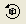enables to change the view-point.

NOTE: as explained in the iData axis definition, the axis of rank 1 is usually assigned to the 'Y' label, and the axis of rank 2 to 'X'.

#### Plots for 3D objects

Objects that contain volumetric data Signal=f(x,y,z) can also be displayed as iso-surfaces or semi-transparent volumes, such as in the following example:
`>> [x,y,z,v]=flow; c=iData(x,y,z,v); ;>> plot(c);`
The default plotting method used is an isosurface using an optimal signal value. Other rendering can be plotted with e.g.:
`>> plot(c,'surf median');    % plots the c=median(signal) isosurface, same as plot(d) [default]>> plot(c,'surf mean');      % plots the c=mean(signal) isosurface>> plot(c,'surf half');      % plots the c=(max-min)/2 isosurface>> plot(c,'plot3');          % plots a volume rendering with semi-transparent style, same as plot3(c)>> plot(c,'scatter3');       % a set of colored points in space, same as scatter3(c)>> plot(c,'waterfall');      % a set of colored points in space, same as contourf(c) and waterfall(c)`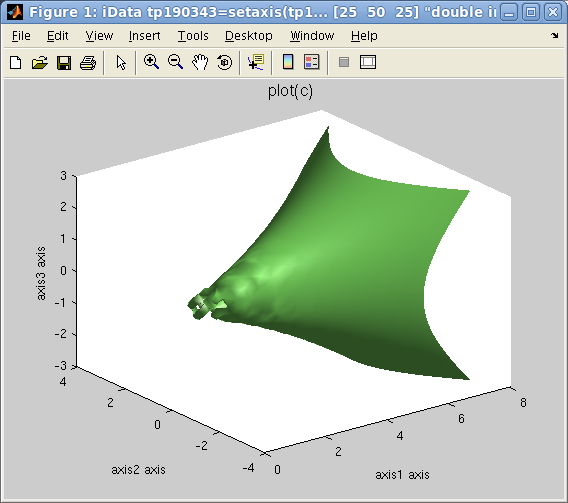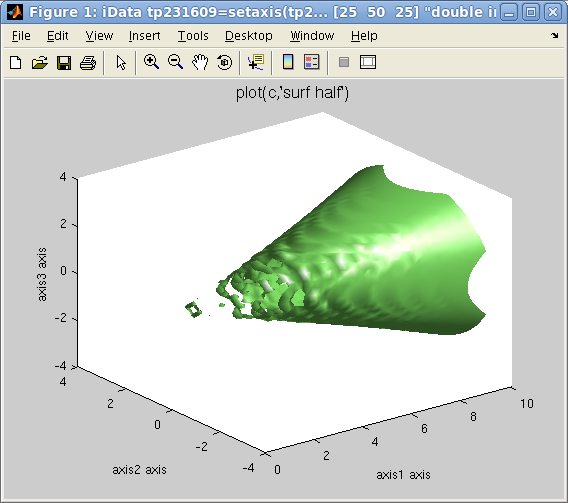Plot 3D: left=plot(d), right=plot(d,'surf half')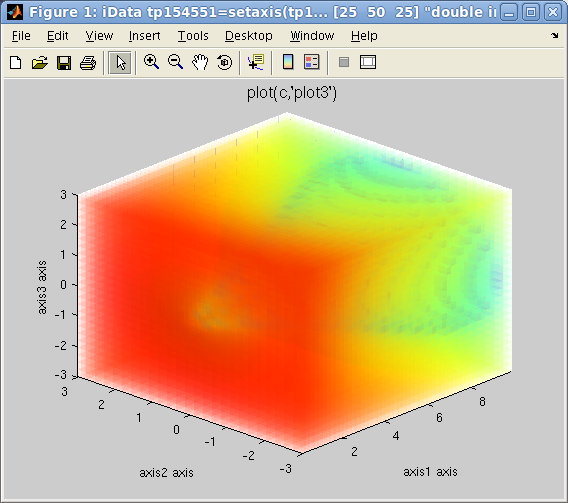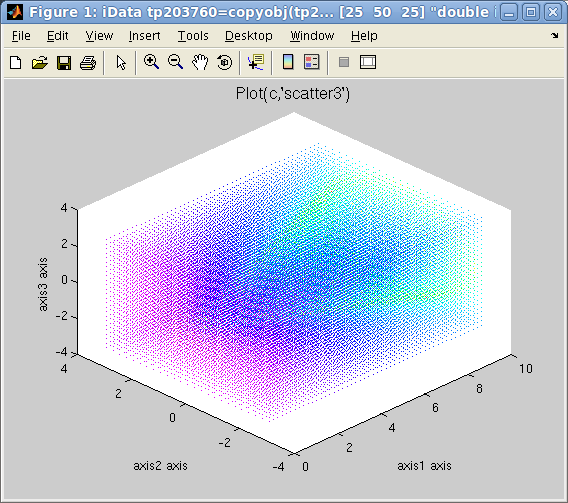left=plot3(d), center=scatter3(d), right=waterfall(d)

It is possible, by right-clicking on the surface or the axis lines, to pop-up a contextual menu which provides the following operations:
• an information dialog about the object (see right image above) and the iFit/iData library version,
• the possibility to duplicate the view into an other window,
• simple way to toggle a grid
• the possibility to smooth the surface appearance, add light and make it semi-transparent. Consecutive light and transparency selections enhance the rendering
• simple way to toggle linear or log scale for the Signal
• simple way to toggle a perspective rendering
• a 'Reset view' item to re-plot the initial object view.
In addition to the editand zoom tools, a rotate view toolenables to change the view-point.

Last but not least the Slice-o-matic viewer for 3D volumetric data set is requested upon command:
`>> slice(c)    % with ndims(c)=3`
Slices and isosurfaces can be viewer with an interactive tool (see below).

NOTE: as explained in the iData axis definition, the axis of rank 1 is usually assigned to the 'Y' label, and the axis of rank 2 to 'X'.

#### Options for 2D and 3D objects

The 2D ans 3D rendering may be customized by mean of keywords added to the second argument to the plot function call, as follows:
• flat, interp, faceted: affects the type of shading used (see help shading).
• transparent, light: adds transparency (see help alpha), and add some light(see help light).
• clabel: requests a labeling of contours, by value (see help clabel)
• axis tight, axis auto: sets plot axis limits tight around the object, or automatically optimized (see help axis)
• view2, view3: displays 2D/3D views from top or oblique view
• painters (bitmap drawing), zbuffer (vectorial drawing): selects the Matlab painters for rendering.
• hide_axes hides XYZ axes and labels, but retains the Title
• shifted for overlayed plots makes sure plots are regularly shifted on the Z axis for better shifted overlayed rendering.
A usage examples is
`>> plot(a,'surf interp view2 axis tight transparent')`

### Plotting an object array in a single plot

#### Overlayed plot

All plot functions can be overlay-ed with the usual hold on Matlab command. However, the iData methods provide an integrated way to plot an array of objects onto the same figure, such as in the following example:
`>> x=-pi:0.01:pi; a=iData(x,x); >> a.Error=0;                         % replace default Error=sqrt(Signal) by no-error.>> b=sin(a); c=cos(a); d=exp(-a.*a);  % create new objects by applying operator on the initial linear one>> plot([a b c d]);                   % overlay all objects`
This can also be applied to 2D and 3D objects, even though the latter usually produces a crowded plot.
`>> [x,y,z]=peaks; a=iData(x,y*10,z); >> c=linspace(a,-a+50,10);            % continuously go from 'a' to a '-a+50' in 10 steps>> plot(c);                           % plot all on the same figure`
which overlays a set of objects, as shown below with a chosen view angle.

`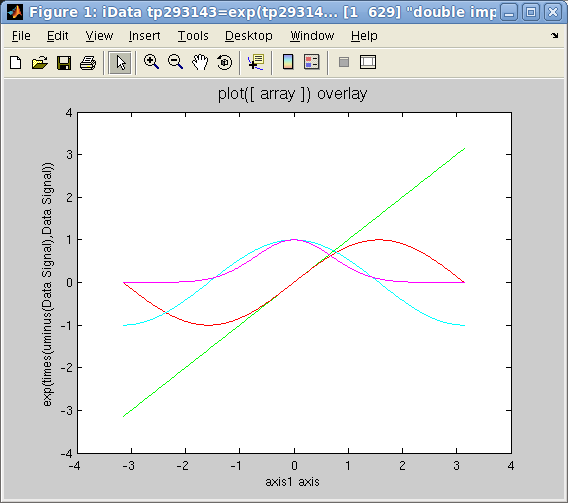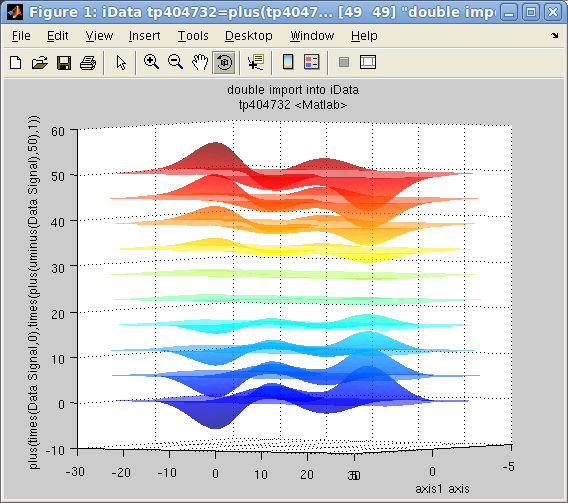`
Plot overlay: plot([ array ]): left=1D, right=2D

Any array of objects can be plotted, including object mix with dimensionalities 1, 2 or 3.

#### Side by side plotting: Single objects and Catenated objects (waterfall)

A common way to plot a set of 1D curves which depend on a parameter is to plot them side by side (waterfall style), so as to form a surface. Let us build an array of 1D objects and assign it an additional axis.
`>> x=-pi:0.01:pi; a=iData(x,x); >> a.Error=0;                         % replace default Error=sqrt(Signal) by no-error.>> b=sin(a); c=cos(a); d=exp(-a.*a);  % create new objects by applying operator on the initial linear one`
The objects we have defined are 1D objects. In order to have them appear in a 2D coordinate frame, one way is to simply assign a new axis to each object, and then display them as a surface:
`>> a{2}=1; b{2}=1.5; c{2}=3; d{2}=5;  % assign a new 2D axis single value to each 1D objects>> plot([a b c d],'surf');            % plot all as a set of lines side by side>> waterfall([a b c d])               % same as above>> surf([a b c d]`
If the X axis (rank 2) has not been defined, the index of the objects in the array is used. The surf method 'surf' and 'scatter3' methods are here equivalent (colored lines), whereas 'plot3' and 'waterfall' draw simple lines.

An other way to achieve a similar result is to assemble the 1D objects into a 2D object, and then define the new axis values:
`>> e=cat(2, [a b c d]);               % catenate 1D objects into a 2D object along 2nd axis >> e{2} = [ 1 1.5 3 5 ];              % assign 2nd axis values in one go>> plot(e,'mesh');                    % plot`
which has the advantage that the new axis is directly assigned to a vector, instead of independent single values.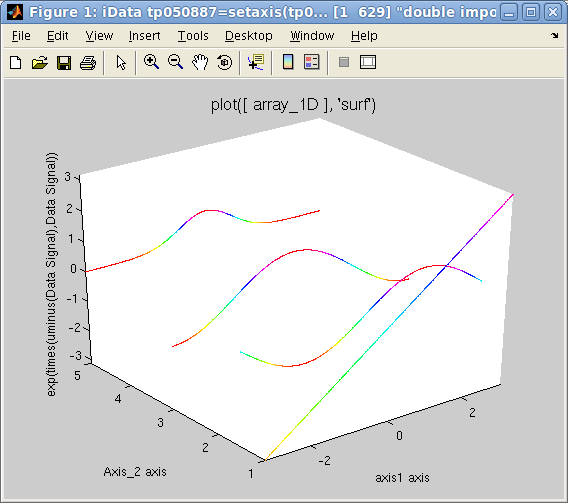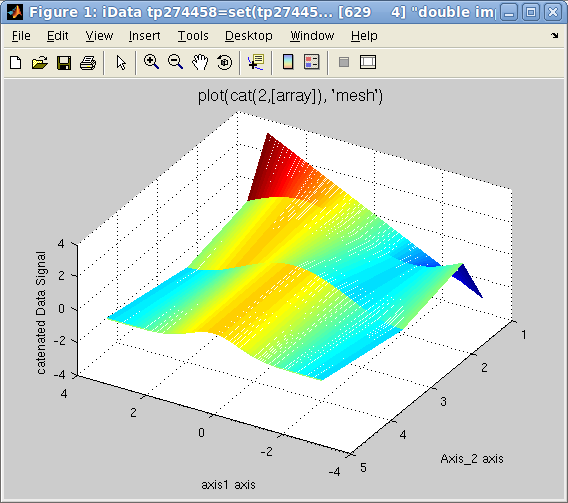Plots side by side: left=separated plots, right=catenated objects

### Plotting object array in a set of panes (multiple-plots aka subplot)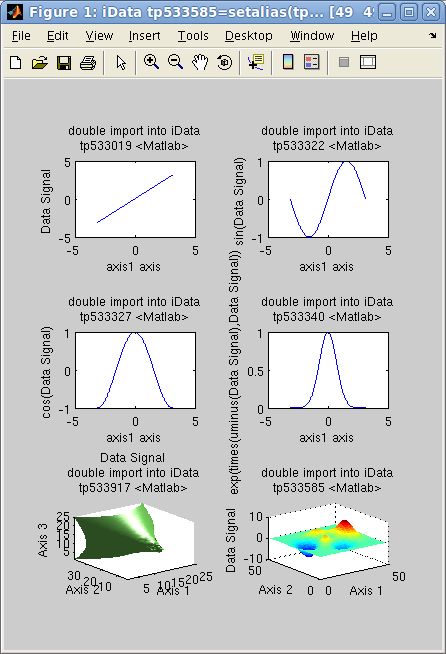In case one wishes to display a number of objects into tiled independent coordinate frames, all gathered into the same window, the subplot function can be used the same way as with the overlay method shown above.
`>> x=-pi:0.01:pi; a=iData(x,x); a.Error=0; % replace default Error=sqrt(Signal) by no-error.>> b=sin(a); c=cos(a); d=exp(-a.*a);       % create new objects by applying operator on the initial linear one>> e=iData(flow); f=iData(peaks);          % create 2D and 3D objects>> subplot([a b c d e f]);                 % plot all into a set of separate frames`
The number of tiles is automatically set to a sensible arrangement but can be set by hand by giving an additional [m n] vector of tile dimensions.
`>> subplot([a b c d e f], [ 2 3 ]); `
Additional arguments can also be given to customize the type of plot rendering:
`>> subplot([a b c d e f], 'mesh tight');>> subplot([a b c d e f], [2 3], 'surf light');  `

### Plotting projections and slices

Portions of an object can equally be displayed with plot and subplot.

#### Plotting projections

The integration operators sum, camproj and trapz can be used to produce data projections.

The sum computes the raw serial sum of the signal along a given dimension. The trapz trapezoidal integration computes the same, but takes additionally into account the axes values. Finally, the camproj does the same as sum, but on all other axes than the one specified. In this respect, camproj always produces a 1D vector which is the projection of the object on the selected dimension.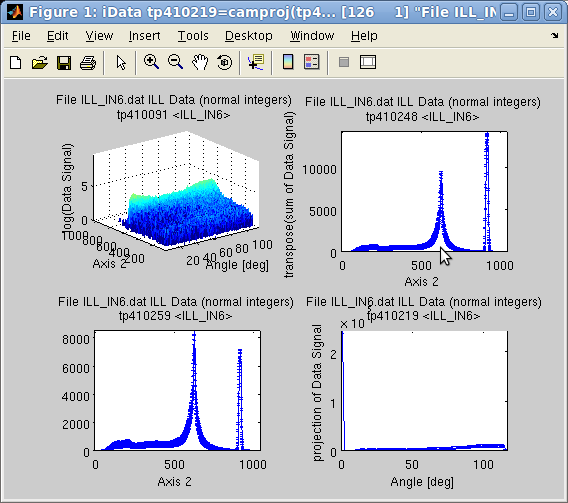On a 2D object sum(a,1) is the same as camproj(a,2) and sum(a,2) is camproj(a,1).
`>> a=iData([ ifitpath 'Data/ILL_IN6.dat' ]);	% import data>> setalias(a, 'Angles', 'Data.FFFFFFFFFFFFFFFFFFFFFFFFFFFFFF_7(32:371)','Angle [deg]');>> a{1} = 'Angles';	% define the angular axis>> xlabel(a,'Time channel');>> setalias(a, 'Wavelength','Data.FFFFFFFFFFFFFFFFFFFFFFFFFFFFF_10(21)','Neutron wavelength [Angs]');>> setalias(a, 'ElasticChannel','Data.FFFFFFFFFFFFFFFFFFFFFFFFFFFFF_10(9)','Elastic channel position [ch]');>> setalias(a, 'ChannelWidth','Data.FFFFFFFFFFFFFFFFFFFFFFFFFFFFF_10(18)','Channel time width [um]');>> setalias(a, 'LSD','Data.FFFFFFFFFFFFFFFFFFFFFFFFFFFFF_10(27)','Sample-Detector Distance [m]');>> subplot([ log(a) sum(a) trapz(a) camproj(a) ],'axis tight');`

#### Plotting slices (orthogonal)

Data slices and subsets can be obtained by selecting subspaces from their corresponding indices.
`>> a=iData([ ifitpath 'Data/ILL_IN6.dat' ]); >> plot(a(:,622));                   % extract the object made from channel 622 on second axis, with all columns`
The sliceomatic viewer is displayed for 3D objects with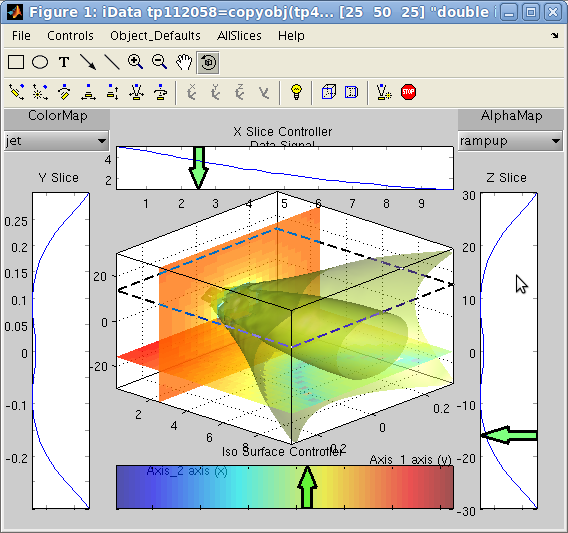`>> a=iData(flow);>> slice(a)`
You can then define the intensity level for the iso-surface (lower colorbar), its transparency (from contextual menus), and create/move slices on 3 axes (side projections). Selections show up as green arrows which have contextual menus and can be moved, changed, removed. You may add as many slices/isosurfaces as you wish. The slices can only be selected along the coordinate frame planes.

Warning: if you use the rotate toolyou must disable it (click again on the tool after rotating the view) to resume the slice edition.

For 2D objects, a similar, yet simpler, tool is displayed. You may use the mouse and arrow keys to move slices, which are shown in separate side windows. You may use the rotate tool to change the orientation. Pressing the Return key exits the tool, but retains the cuts. Closing the data set windows closes all views.

#### Plotting slices (any orientation) - select region of interest (ROI)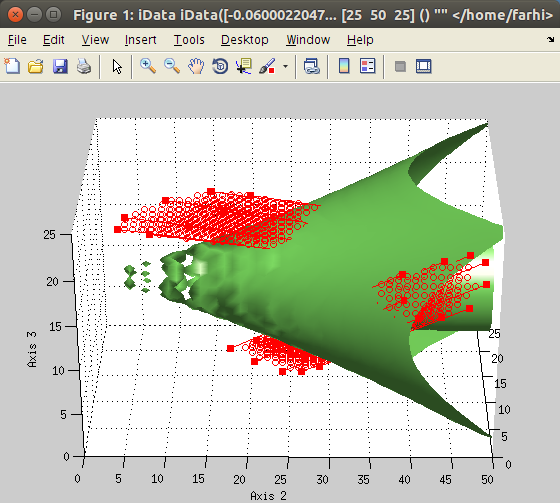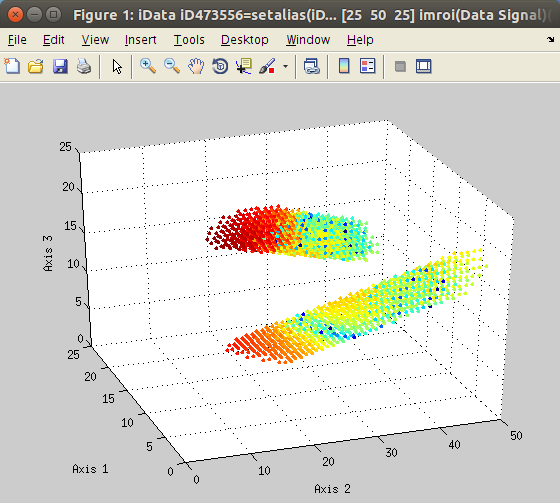The imroi selection tool allows to select polygonal regions of interest (ROI, left).
Once extracted, the new data set can be plotted (right).

The imroi method allows to define interactively a set of points on a view. These points are then used to form a convex hull from its vertices. The intersection of these areas with the data set defines the regions of interest (ROI).
`>> a=iData(flow);>> [b, mask, h] = imroi(a);>> figure; scatter(b);	% plot the selection>> close(h); 		% close the ROI tool view`
You may undo (remove) previous points/lines from the active polygon by pressing the mouse right-button or the BackSpace key.
To clear the current ROI and start over, press the 'c' or DEL key. It is possible to define as many separate ROI's by pressing the 'a' key to add to store the current polygon, and start a new one.

When used with surfaces and volumes, you may rotate the view by pressing the 'r' key over the figure. This stores the current polygon definition, and switch to the rotate mode. Once the view is properly oriented, disable the Rotate tool (click again on the rotate icon) to enter a new polygon definition. An easier way, using +/- 5 deg steps, is to use the up/down/left/right arrow keys.

Once all ROI's are defined, press the middle mouse button or the Return key to end the ROI selection and compute the intersection with the data set. To abort (cancel) and exit the ROI tool, use the Escape key.

After the ROI have been selected, you may close the data set view, or check visually the ROI's.

 Action / key (case insensitive) What it does mouse left click or SPACE add a point/line to the current ROI polygon right-click or BACKSPACE remove last point middle-click or Q or RETURN terminate input and compute the intersections (quit) DEL or C remove all points (clear) A store the current ROI and start a new separate polygon (add) R switch to the rotation mode. Click again onto switch back to the ROI definition with a new polygon. ESC abort (exit immediately) H display a Help dialogue up/down/left/right arrow rotate the view by +/- 5 deg
imroi: interactive actions

The resulting object is a copy of the initial one, but only retaining the selected areas, the rest being set to NaN. In addition, it is possible to retrieve the 'mask' which is a data set with 1 where the ROI's apply, and 0 elsewhere (unselected areas). You may multiply this mask with any data set to apply it.

You may customize the imroi plotting style by adding a 2nd argument which is passed to the plot method, e.g.
• b = imroi(a, 'plot3');

### Setting plot options: title, axes labels, axes limits

Even though it is possible to set the labels on a Matlab figure with the title xlabel ylabel zlabel functions, it is possible to store that information into the object, so that further plots show it. It is also possible to extract portions of an object by specifying the axes limits.

#### Setting labels, title

To set the Signal label, use
`>> title(a, 'a new Signal label')`
which is different from the object title a.Title which holds the name of the whole data set, independently of the assigned Signal in the Data. Also, the object may be assigned a global label a.Label which may be used e.g. to store object categories (background, calibration, with sample, ...).
The Signal label can be obtained with
`>> title(a)>> label(a,0)>> label(a,'Signal')`
Similarly, the axes can be assigned labels with
`>> xlabel(a, 'a new X axis label');              % second axis (columns) ,or 1st axis for 1D objects>> ylabel(a, 'a new Y axis label');              % first axis (rows)>> zlabel(a, 'a new Z axis label');              % third axis>> clabel(a, 'a new C axis label');              % fourth axis>> label(a, rank, 'a new axis label for rank');  % any axis of given rank=1, 2, 3, ..., 0 for signal`
All of these will be shown upon call to plot(a). The values can be obtained with e.g
`>> xlabel(a)>> label(a, rank)>> ...`

#### Setting axes limits

It is possible to select a subregion of an object by specifying limits among the axes. The resulting object does not hold the outer limits data anymore.
`>> xlim(a,[xmin xmax])`
and similar functions ylim, zlim, clim. Keep in mind that 'X' is along the second axis rank, and 'Y' is along the first.
In order to only affect the view, we rather recommend to use the standard Matlab commands xlim([xmin xmax]) and similar ylim, zlim and clim.

### Exporting plots as figures and images

The figures can be exported from their File/Save as menu item. However, it is possible to automate the export process by directly calling
`>> saveas(a, filename, format)`
where format may be jpg, png, pdf, HDF ... All formats are detailed in the Save page.
The getframe method generates a snapshot picture (thumbnail).

### Importing plots as iData objects

As seen in the Load documentation page, the iData method can be used with graphical object including figure and axis handle arguments.
`>> a=iData(gcf);`

E. Farhi - iFit/iData plotting - Nov. 27, 2018 2.0.2 - back to Main iFit Page